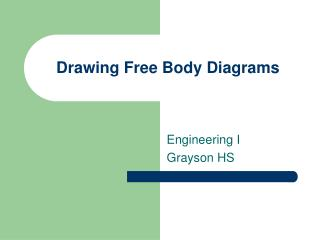# Drawing Free Body Diagrams - PowerPoint PPT PresentationDownload PresentationDrawing Free Body Diagrams

Drawing Free Body Diagrams
Download Presentation## Drawing Free Body Diagrams

- - - - - - - - - - - - - - - - - - - - - - - - - - - E N D - - - - - - - - - - - - - - - - - - - - - - - - - - -
##### Presentation Transcript

1. Drawing Free Body Diagrams Engineering I Grayson HS

2. Free Body Diagrams • Free-body diagrams are diagrams used to show the relative magnitude and direction of all forces acting upon an object in a given situation. • a special example of a vector diagram.

3. Free Body Diagrams • The size of the arrow in a free-body diagram is reflects the magnitude of the force. • The direction of the arrow shows the direction which the force is acting. • Each force arrow in the diagram is labeled to indicate the exact type of force. • It is generally customary in a free-body diagram to represent the object by a box and to draw the force arrow from the center of the box outward in the direction which the force is acting.

4. Free Body Diagrams • The only rule for drawing free-body diagrams is to depict all the forces which exist for that object in the given situation. • to construct free-body diagrams, it is extremely important to know the various types of forces. Basic Forces are: • Gravity • Normal Forces (push of earth up on a load) • Thrust • Friction • Tension • Compression • Torque • Shear

5. Free Body Diagrams • If given a description of a physical situation, begin by using your understanding of the force types to identify which forces are present. • Then determine the direction in which each force is acting. • Finally, draw a box and add arrows for each existing force in the appropriate direction; label each force arrow according to its type. • If necessary, refer to the list of forces and their description in order to understand the various force types and their appropriate symbols.

6. Free Body Diagrams • 1. A book is at rest on a table top. A free-body diagram for this situation looks like this:

7. Free Body Diagrams • 2. A girl is suspended motionless from the ceiling by two ropes. A free-body diagram for this situation looks like this:

8. Free Body Diagrams • 3. An egg is free-falling from a nest in a tree. Neglect air resistance. A free-body diagram for this situation looks like this:

9. Free Body Diagrams • 4. A flying squirrel is gliding (no wingflaps) from a tree to the ground at constant velocity. Consider air resistance. A free-body diagram for this situation looks like this:

10. Free Body Diagrams • 5. A rightward force is applied to a book in order to move it across a desk with a rightward acceleration. Consider frictional forces. Neglect air resistance. A free-body diagram for this situation looks like this:

11. Free Body Diagrams • 6. A rightward force is applied to a book in order to move it across a desk at constant velocity. Consider frictional forces. Neglect air resistance. A free-body diagram for this situation looks like this:

12. Free Body Diagrams • 7. A college student rests a backpack upon his shoulder. The pack is suspended motionless by one strap from one shoulder. A free-body diagram for this situation looks like this:

13. Free Body Diagrams • 8. A skydiver is descending with a constant velocity. Consider air resistance. A free-body diagram for this situation looks like this:

14. Free Body Diagrams • 9. A force is applied to the right to drag a sled across loosely-packed snow with a rightward acceleration. A free-body diagram for this situation looks like this:

15. Free Body Diagrams • 10. A football is moving upwards towards its peak after having been booted by the punter. A free-body diagram for this situation looks like this:

16. Free Body Diagrams • 11. A car is coasting to the right and slowing down. A free-body diagram for this situation looks like this:

17. Free Body Diagrams • Exercise: • Draw a free body diagram for the CAD Lab television that is hanging on the wall. • Draw a free body diagram for the flag in the front of the class. • Draw a free body diagram for the book cart being pushed from the back of the room to the front

18. Free Body Diagrams • http://www.glenbrook.k12.il.us/GBSSCI/PHYS/Class/newtlaws/u2l2c.html